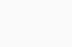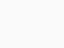# Change the size of a numpy array in Python

In this article, we will learn how to change the size of a numpy array in Python.

First, let’s understand what a numpy array is.
A NumPy array is a part of the NumPy library which is an array processing package.

```import numpy as np
eg_arr = np.array([[1,2],[3,4]])
print(eg_arr)```

Run this code online
Using np.array, we store an array of shape (2,2) and size 4 in the variable eg_arr.Now, let’s see how can we change the size of the array.

## Changing size of numpy Array in Python

Size of a numpy array can be changed by using resize() function of the NumPy library.

numpy.ndarray.resize() takes these parameters-

1. New size of the array
2. refcheck- It is a boolean that checks the reference count. It checks if the array buffer is referenced to any other object. By default, it is set to True. You can also set it to False if you haven’t referenced the array to any other object.

During resizing, if the size of the new array is greater than the given size, then the array is filled with 0’s. Also, it resizes the array in-place.

Now let’s understand it with some examples.

## Changing size of array with numpy.resize()

Example 1 –

```import numpy as np
cd = np.array([2,4,6,8])
cd.resize((3,4),refcheck=False)
print(cd)```

Run this code online

The resize function changes the shape of the array from (4,) to (3,4). Since the size of the new array is greater, the array is filled with 0’s.
So this gives us the following output-Example 2 –

```import numpy as np
cd2 = np.array([[1,2],[3,4]])
cd2.resize((5,6),refcheck=False)
print(cd2)```

Run this code online

The resize function changes the array from (2,2) to (5,6) and fills the remaining portion of the array with 0’s.
Here’s the output-```import numpy as np
cd3=np.array([[1,2],[3,4]])
cd3.resize((2,1),refcheck=False)
print(cd3)```

Run this program online

Here, the size of the new array is smaller, so this gives the following output-I hope you all liked the article!